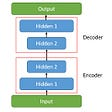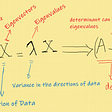# Student’s marks prediction using python

In these era of machine learning and artificial intelligence we can now predict the marks of a student which is to be achieved in the next semester.

These will help teachers with the student’s performance. Teacher can ask their students to improve on a particular subject so that students can improve their performance.

Main objective is to help teachers analyze students performance easily.

Let’s move on where we get our hands dirty with the python.

Dataset used here is the UCI dataset of a portugese schools of secondary education student. Link of the dataset: https://archive.ics.uci.edu/ml/datasets/student+ performance#

I have used 4 regression techniques which are as follows:

# Linear Regression

Linear regression is used for finding linear relationship between target and one or more predictors. There are two types of linear regression- Simple and Multiple.

Advantages: Linear Regression is simple to implement and easier to interpret the output coefficients.

Disadvantages:On the other hand in linear regression technique outliers can have huge effects on the regression and boundaries are linear in this technique.

# Random Forest Regressor

A random forest is a meta estimator that fits a number of classifying decision trees on various sub-samples of the dataset and uses averaging to improve the predictive accuracy and control over-fitting.

Advantages: Random Forest can be used to solve both classification as well as regression problems.

Disadvantages: Random Forest require much more time to train as compared to decision trees as it generates a lot of trees (instead of one tree in case of decision tree) and makes decision on the majority of votes.

Gradient Boosting is similar to AdaBoost in that they both use an ensemble of decision trees to predict a target label. Calculate the average of the target label. Calculate the residuals residual = actual value — predicted value.
Construct a decision tree
Predict the target label using all of the trees within the ensemble

Advantage: Lots of flexibility — can optimize on different loss functions and provides several hyperparameter tuning options that make the function fit very flexible.

Disadvantages: The high flexibility results in many parameters that interact and influence heavily the behavior of the approach (number of iterations, tree depth, regularization parameters, etc.). This requires a large grid search during tuning.

Bayesian Ridge

In the Bayesian viewpoint, we formulate linear regression using probability distributions rather than point estimates. The response, y, is not estimated as a single value but is assumed to be drawn from a probability distribution.

The aim of Bayesian Linear Regression is not to find the single “best” value of the model parameters, but rather to determine the posterior distribution for the model parameters.

Advantages: It’s good when you have a linear regression problem and want to use a Bayesian approach.

Disadvantages: It’s not great when you don’t have a regression problem, or if a linear model does not work well, or if you do not want a Bayesian approach.

These techniques are used to achieve more accurate result.

# Exploratory data analysis

Import the necessary libraries.

import numpy as np
import pandas as pd
import matplotlib.pyplot as plt

To see what our data set looks like use head function as shown below:

Now define the variables which can are more varying in the dataset. Here I have taken these following variables:

Further on we will divide the dataset into training and testing dataset. Using sklearn.

# Train the model:

## Bayesian Ridge

After all these regression its time to find the accuracy of the model and predict the marks of the student.

Here the accuracy is 73%, which means that whatever prediction will be done will be 73% accurate.

These accuracy is achieved by using ensemble model accuracy as shown in above figure.

## More from NISARG SATANI

Cloud and DevOps Enthusiast

Love podcasts or audiobooks? Learn on the go with our new app.

## It's here again, another new week and the weekly FAQ session will still hold.## Autoencoder network optimization for dimensionality reduction## Is the YouTube Dislike Button Necessary?## How Two College Students Made \$600,000 in 24 Hours## Basic Introduction to Pandas: Pandas Series(Part 2)## Sonifiying Everything, A Stained-Glass Space Shuttle, And The “False Banana” Considered As A…## USER-USER Collaborative filtering Recommender System in Python## Is Data Science New……………?## NISARG SATANI

Cloud and DevOps Enthusiast

## A Technical Review of PCA and its Applications: Calculating Similarity of Two Datasets## How to Classify Different Dialects of English